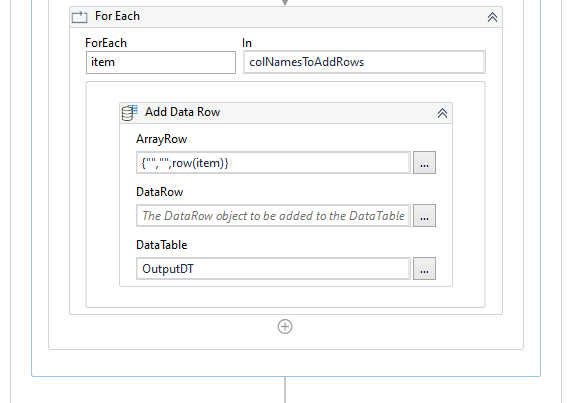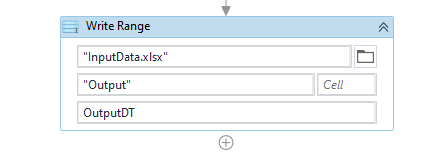# Insert Rows

I have an excel sheet with 1000 rows. I want to insert 9 rows below every row and
I also need to transpose selected column data to the newly added row. Any idea on how to do this using UiPath.

I’ve tried adding new rows but its not added. Is this flow correct?

Hi @Chendoran_M ,

Could you maybe provide us with the Sample Input data and the Expected Output for this data ?

It would be easier for us to analyze and suggest an approach towards creating the solution.

InputData.xlsx (9.6 KB)

Could you check with the below Steps and Implementation :

1. Read the excel sheet as a Datatable using `Read Range` Activity. Create the Output Datatable schema by cloning the Input datatable. Next, as we would require to convert certain column data to rows, store those column names in an array. An Image representation is shown below :

2. Next, Loop through the Input datatable using `For Each Row` Activity. Inside the For Each Row, use `Add Data Row` Activity and Add the First row to the Output Datatable as shown in the image below. The ArrayRow Expression uses the First 3 Column data as it is and then appends empty values to the next column data until the Status Column. Then the Status Column data is appended to it.

3. Next, Adding/Converting of the column data to rows is done using a `For Each` Loop. We use the column names stored to access the values one by one and use `Add Data Row` Activity to add the column values as row data. Since we only need the Prod_Id_* column data we can keep the other columns as Empty data.4. We can then write the `OutputDT` value to an Excel sheet using `Write Range` Activity.Check the workflow below :
Excel_SplitRows_ToMultipleRows.zip (10.0 KB)

I’ve tried, but I could not find the output. The data are not written in excel.

Could you show us what was the implementation done ? Also do note that a workflow was provided in the above post.

Data.xlsx (10.7 KB)
This is the Input and Output files.
How can I implement this using UiPath

A similar logic that was applied to the previous input data could be also applied to this new data. Here, we would need to understand the number of columns that would be available for one column data, i.e in the data we have the column A_MM_ repeated 4 times indicated by the suffix number. Proceeding according to this logic, we would have to add the rows so many number of times as the column numbers repetition.

Check the updated workflow below :
Excel_SplitRows_ToMultipleRows.zip (12.2 KB)

Let us know if you are still facing issues.

Thank you @supermanPunch .

1 Like

This topic was automatically closed 3 days after the last reply. New replies are no longer allowed.